# Subtracting - 5th grade (10y) - math problems

#### Number of problems found: 100

• Numbers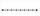Find number on thenumber line that has same distance from this numbers:
• Integers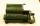May be the sum of two integers less than their difference?
• A number 2A number decreased by the difference between four and the number.
• How manzBy how many are the product of the numbers 328 and 7 greater than their sum?
• Pounds3 pounds subtract 1/3 of a pound.
• Opposite numbers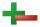Calculate opposite numbers (additive inverse) to given ones:
• Numbers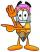By how many is the difference of numbers 8 and 34 less than its product?How much and how many times is 72.1 greater than 0.00721?
• Operations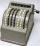Sum of the numbers 1.01 and 3.35 multiply by the difference of numbers 6.69 and 1.39.
• Storm 3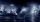If the temperature yesterday was 56 and today is 13 degrees cooler, what is today's temperature?
• What is missing (1000)What number is to add to get 1000?
• Subtraction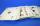How many times you can subtract the number 4 from the number 64?
• Evaluate expressionIf x=2, y=-5 and z=3 what is the value of x-2y
• Identity123456789 = 100 Use only three plus or minus characters to correct previous identity/equation.
• SavingMom said that Suzan saves about 120 EUR (rounded to tens). How many euros could she at least save (minimum)?
• Neighbor angle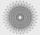For 136° angle calculate size of adjacent angle on one side of a straight line.
• TheatreThe theatrical performance started at 15:50 and ended at 18:50. How many minutes long?
• Expression 6Evaluate expression: -6-2(4-8)-9Add and write the result again as hours, minutes, seconds: 2hodiny45min15s + 1h20m50s =
• Minutes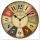Determine the difference in minutes: T1 = 2 3/20 h T2 = 2.3 h

Do you have an interesting mathematical word problem that you can't solve it? Submit a math problem, and we can try to solve it.

We will send a solution to your e-mail address. Solved examples are also published here. Please enter the e-mail correctly and check whether you don't have a full mailbox.

Please do not submit problems from current active competitions such as Mathematical Olympiad, correspondence seminars etc...